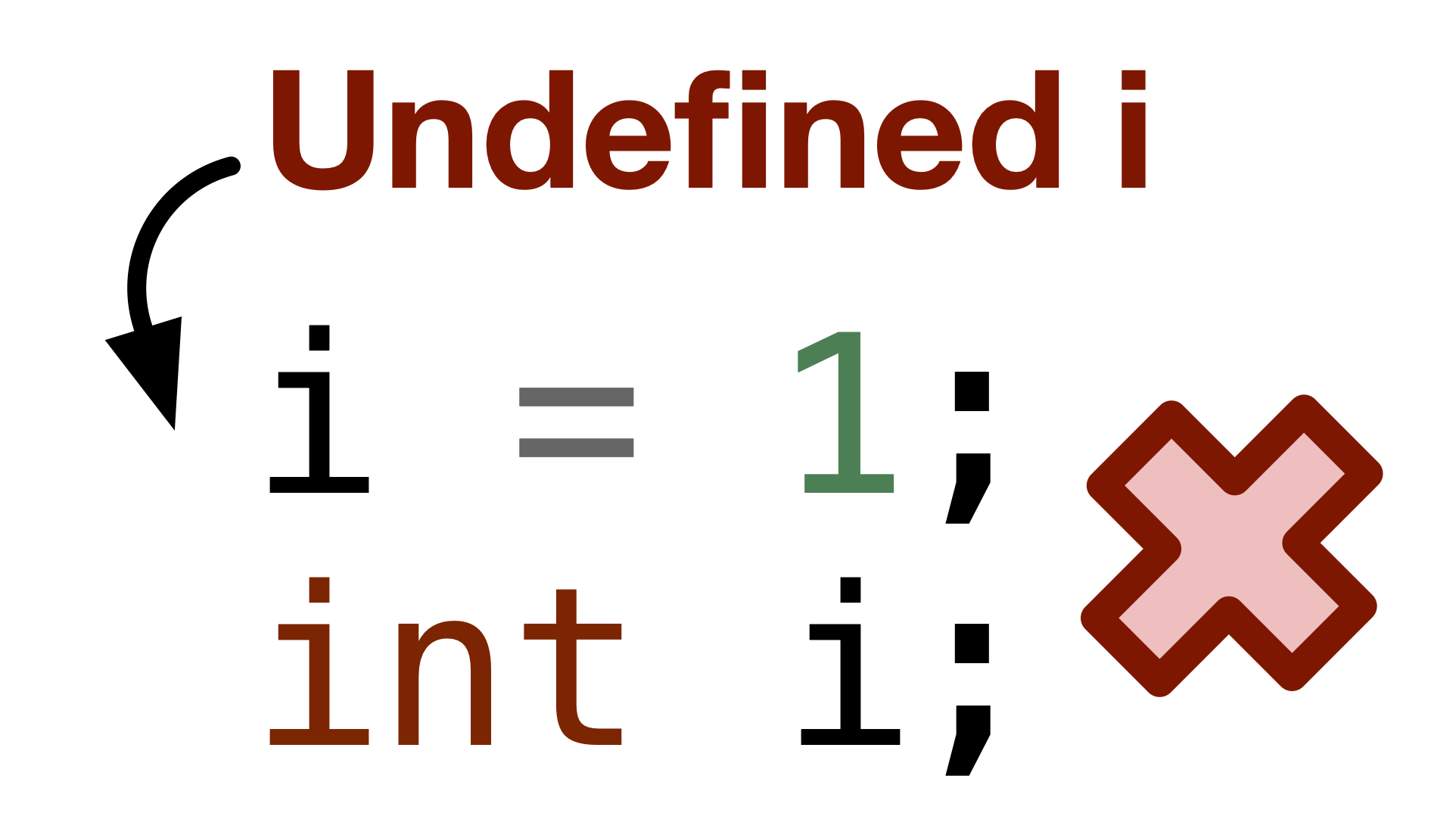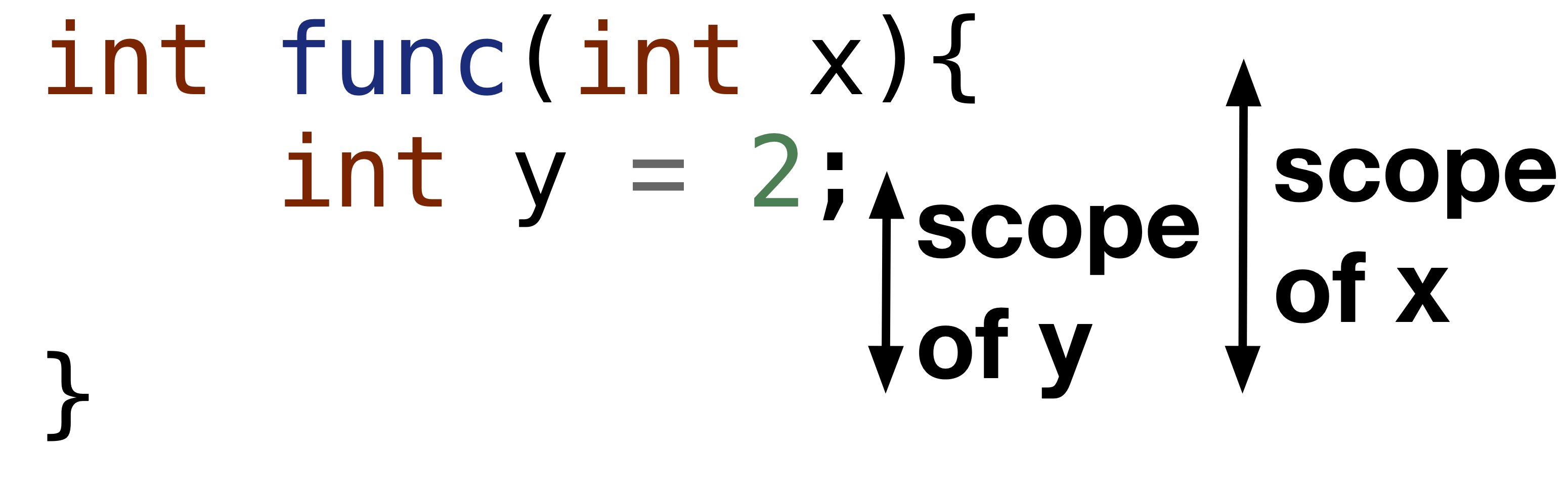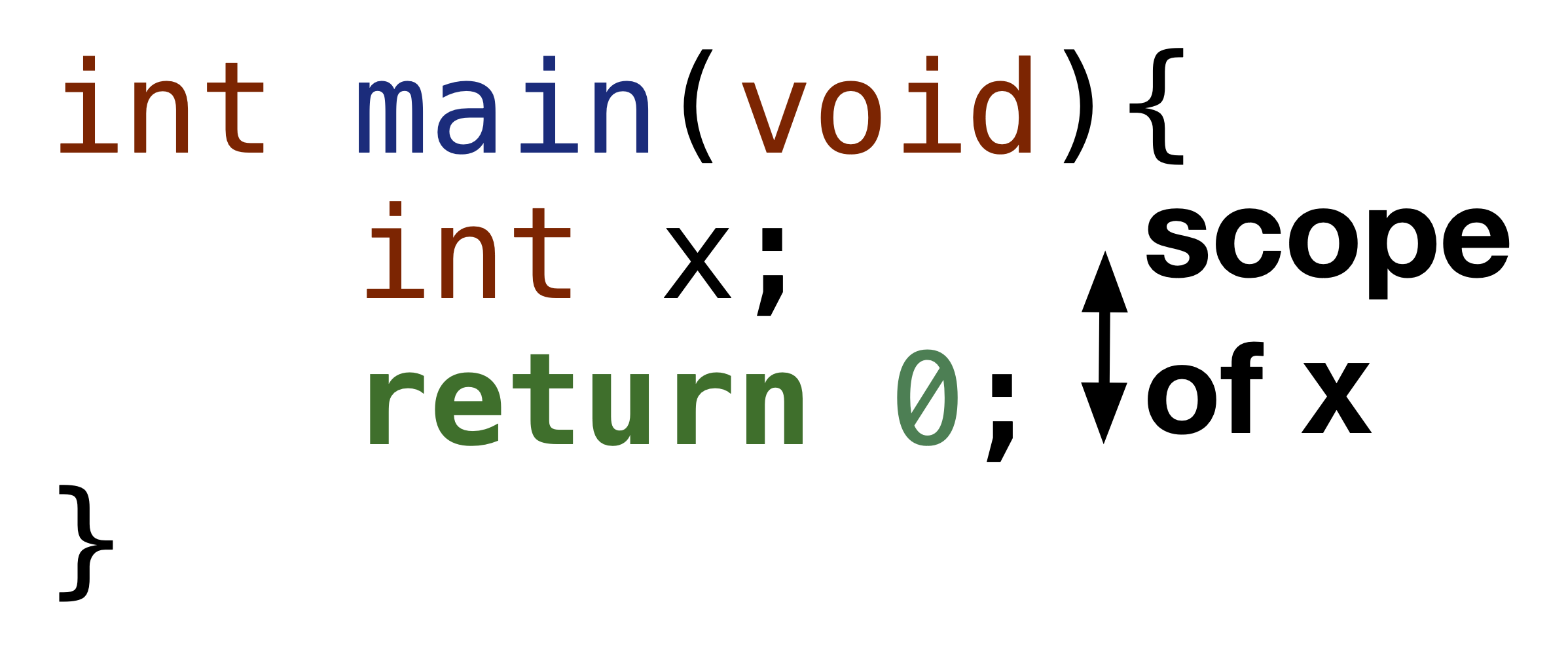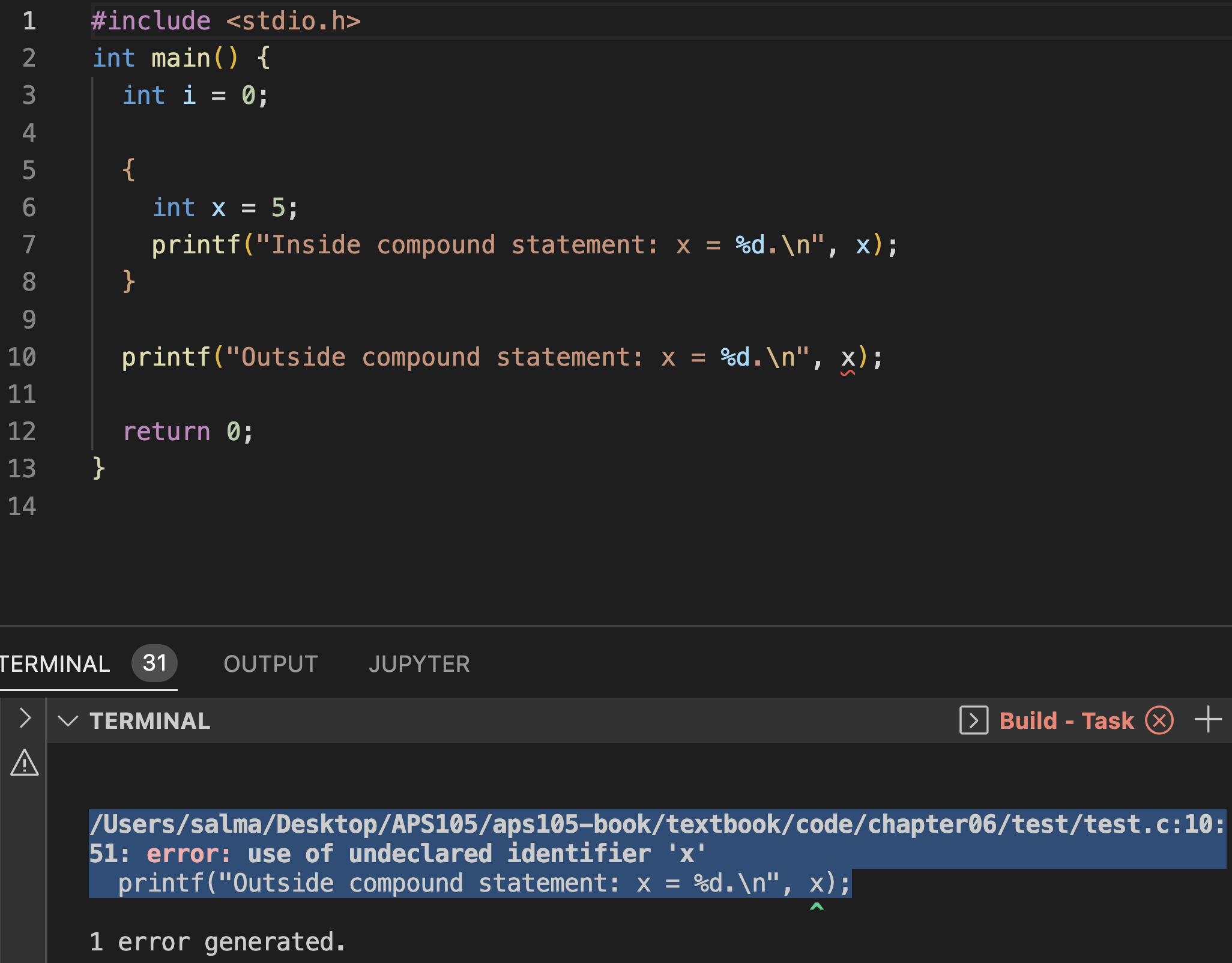# 6.4. Rules defining scope of variables#

Given that now we understand the basics of the C programming language, especially loops, functions and pointers, we can discuss the topic of “scope” in details. The scope of a variable is the set of C statements where the variable is defined/visible/usable. In this section, we cover (revise) the cases where variables have limited scope.

## 6.4.1. Variables can only be used after they are declared.#

For example, the error you will get if you run the following code is “error: use of undeclared identifier ‘i’”.Fig. 6.8 Using i variable before declaring it is not allowed, and will cause compile time error as i is undefined at i = 1; line.#

Code


int main() {
i = 0;
int i;

return 0;
}



Variables declared in a function, can only be used in that function. These variables are referred to as local variables or internal identifiers.

For example, in Fig. 6.9 and Fig. 6.10, we observe the scope of the variables start from the moment they get declared till only the end of the function, even the main function.Fig. 6.9 The scope of x and y in func function starts from where they get declared till the end of the function.#Fig. 6.10 The scope of x in main function starts from where it gets declared till the end of the function.#

## 6.4.2. Use a variable declared in a compound statement#

If you declare a variable within {}, also referred as within a compound statement, and use the variable after the } closing bracket, it is undefined. In other words, the scope of a variable is defined only within the closest {}. For example, the following figure shows a code that uses a variable outside its scope, which is within {}.Fig. 6.11 Using x variable outside the compound statement where it was declared. This causes a compile time error as x is undefined at line 10.#

Code causing compile-time error


#include <stdio.h>
int main() {
int i = 0;

{
int x = 5;
printf("Inside compound statement: x = %d.\n", x);
}
//  Causes compile-time error as x is undefined
printf("Outside compound statement: x = %d.\n", x);

return 0;
}



## 6.4.3. External identifiers/global variables#

The external identifiers or global variables are defined at the top of the .c file outside the main function, and all other functions. Its scope is the entire program. However, it is not good practice to use them, as it can be error-prone. If several functions in your program are using the global variable, and each function is changing it can be difficult to manage/track the value of this global variable. Hence, it is not advisable to use global variables.

Code


#include <stdio.h>

int i = 0;

void func();

int main(void) {
printf("In main: Global variable i = %d.\n", i);
func();
printf("In main after calling func: Global variable i = %d.\n", i);
return 0;
}

void func() {
printf("In func: Global variable i = %d.\n", i);
i = 5;
}



## 6.4.4. Overlapping scope#

You can have a variable with the same name that has an overlapping scope. For example, the following code has two i variables. Their scope is illustrated in the following figure.

Code


#include <stdio.h>

int main(void) {
int i = 1;
printf("Outer i = %d.\n", i);
{
int i = 2;
printf("Inner i = %d.\n", i);
}
printf("Outer i = %d.\n", i);

return 0;
}



## 6.4.5. Practice problem#

In the following figure, we show the scope of different variables in a function. Check your knowledge looking at this figure.